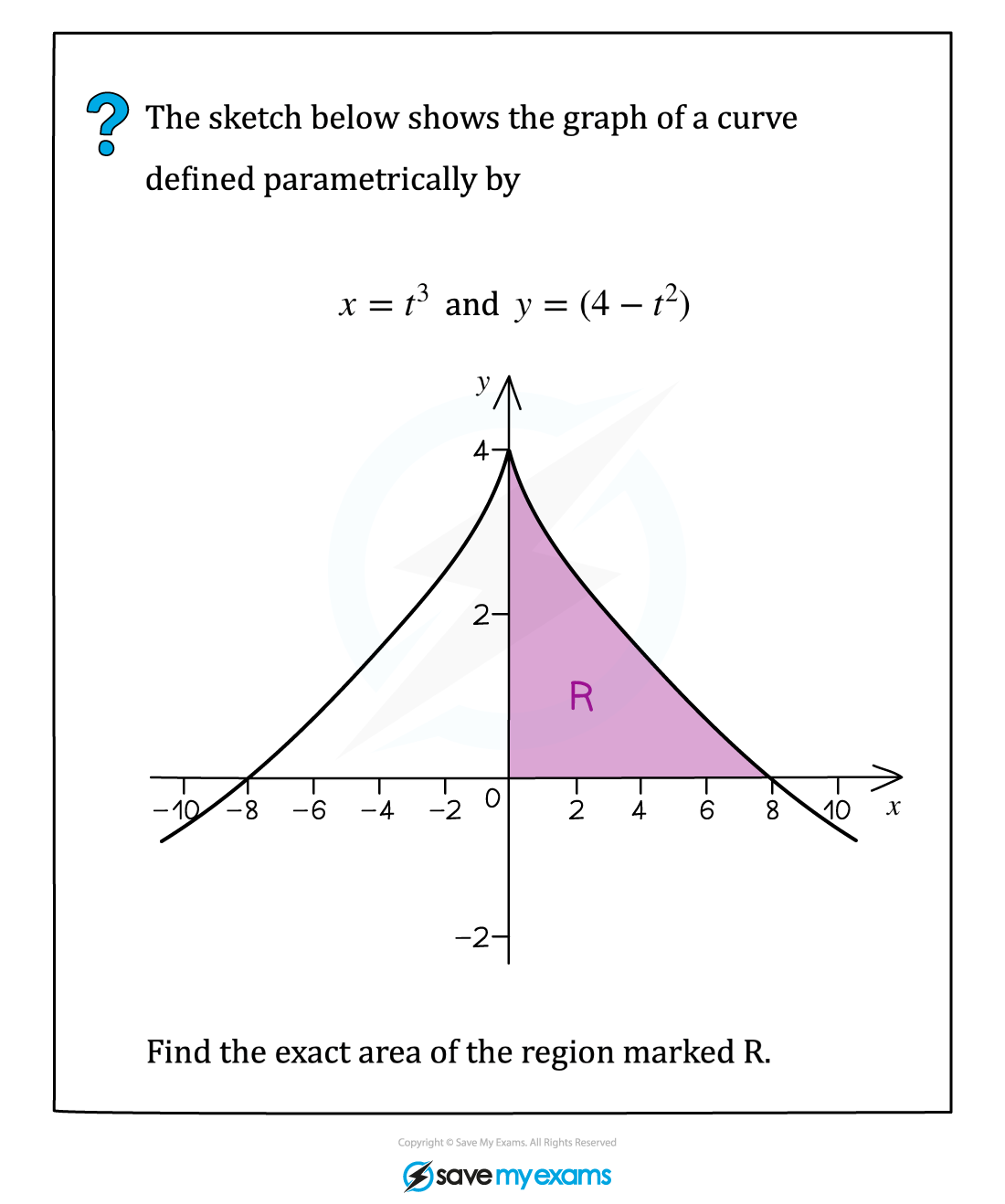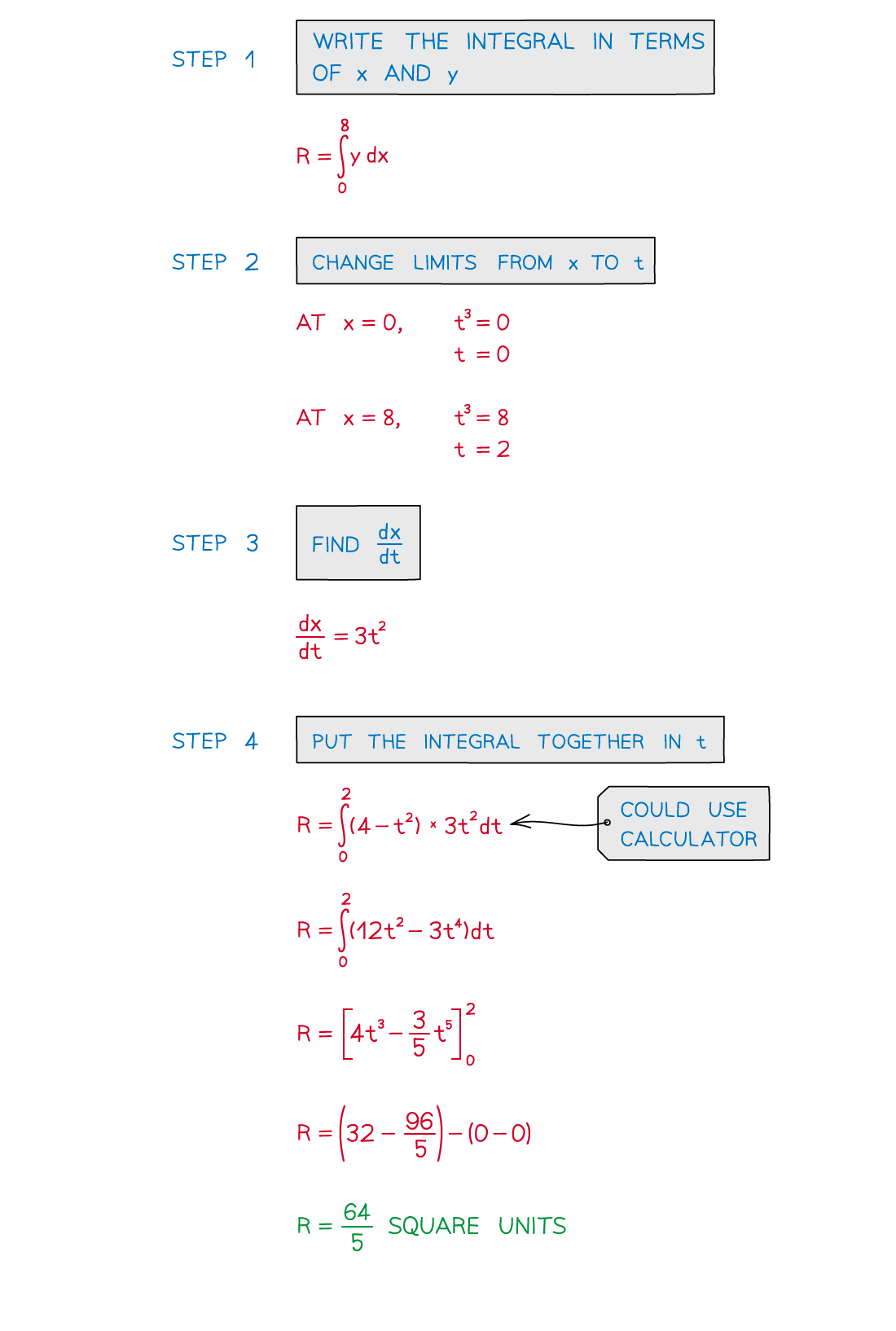# AQA A Level Maths: Pure复习笔记9.2.2 Parametric Integration

### Parametric Integration

#### How does integration work with parametric equations?

• Integration is used to find the area under a curve where the curve has been defined by parametric equations
• x = f(t)
• y = g(t)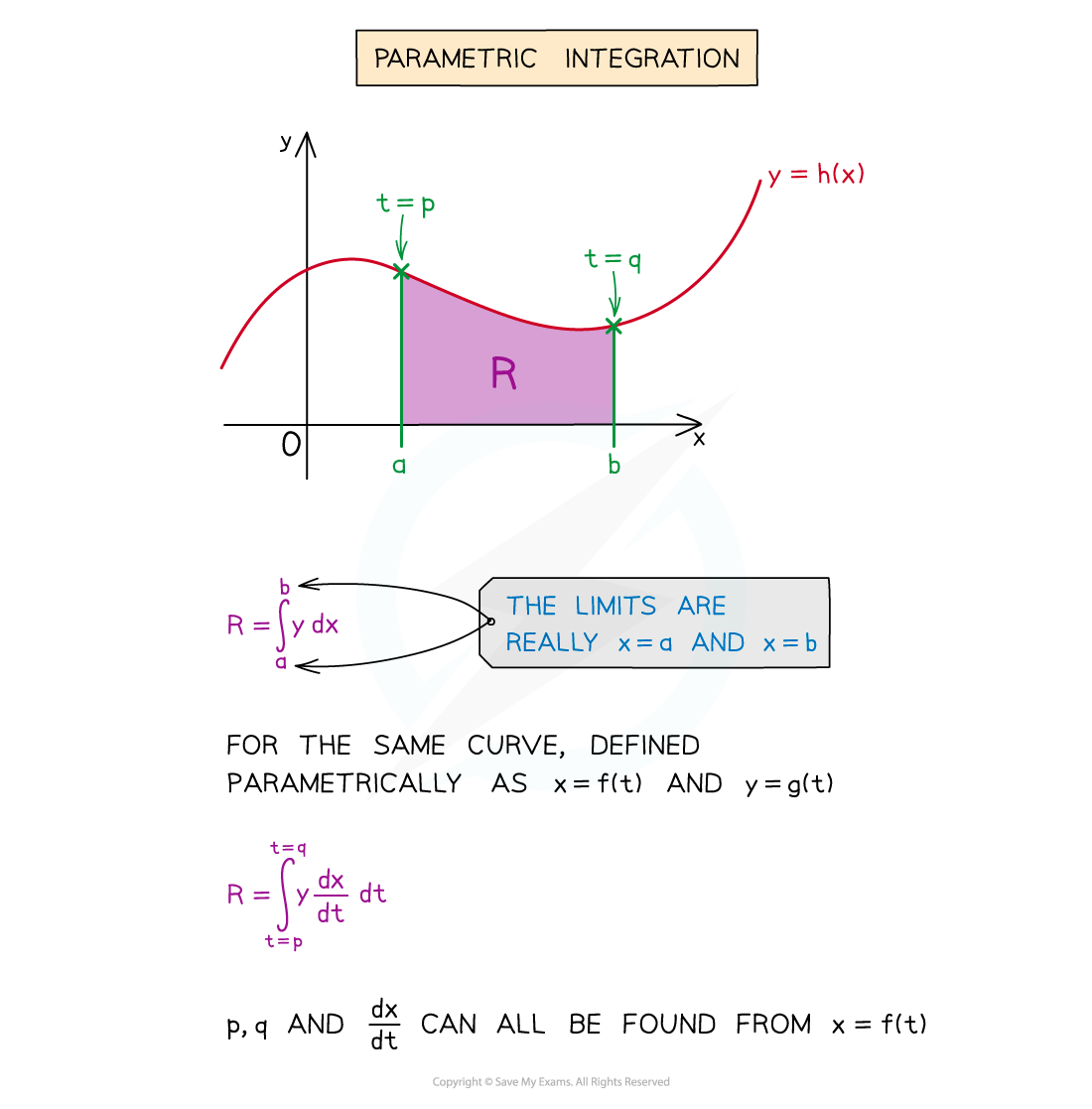• The key point is to ensure the limits of the integral are changed to the parameter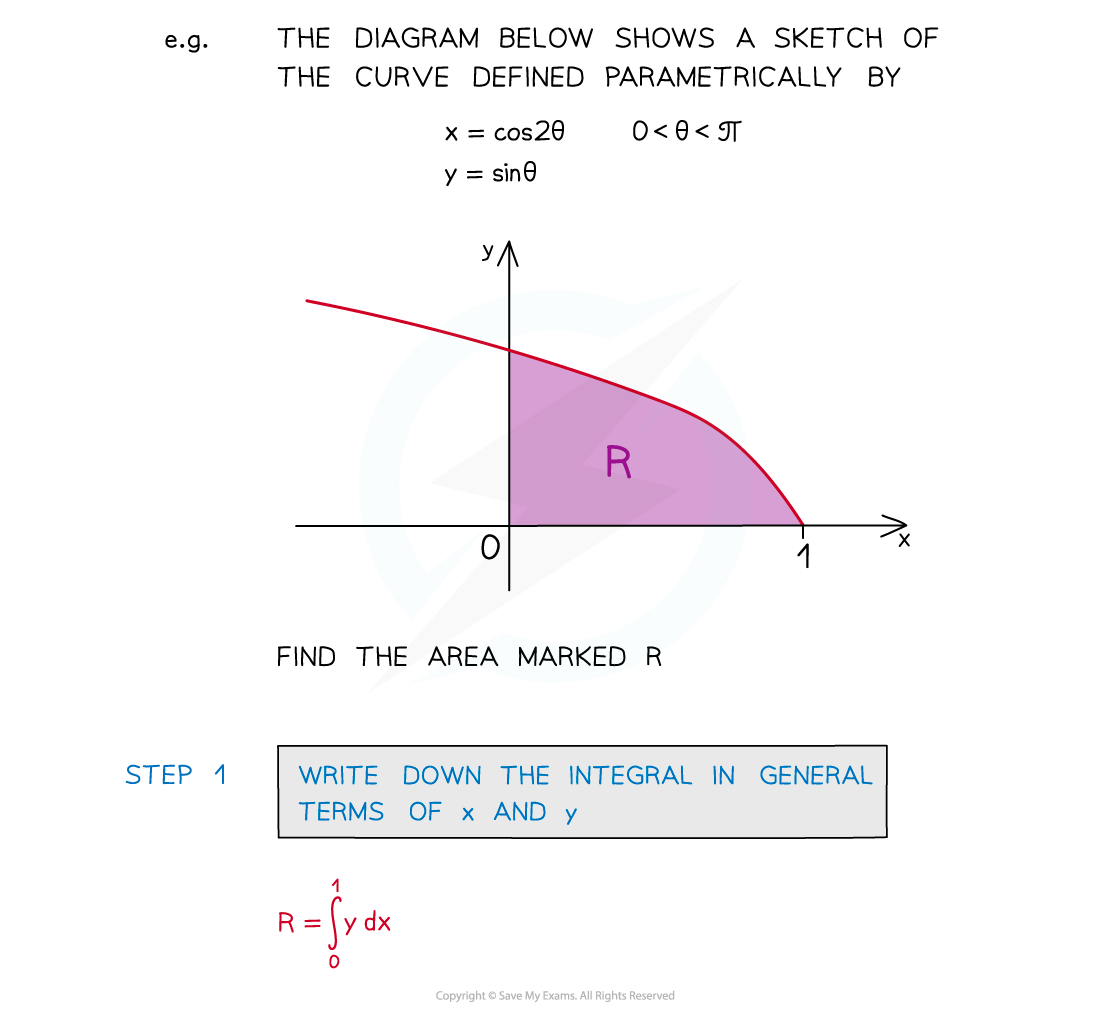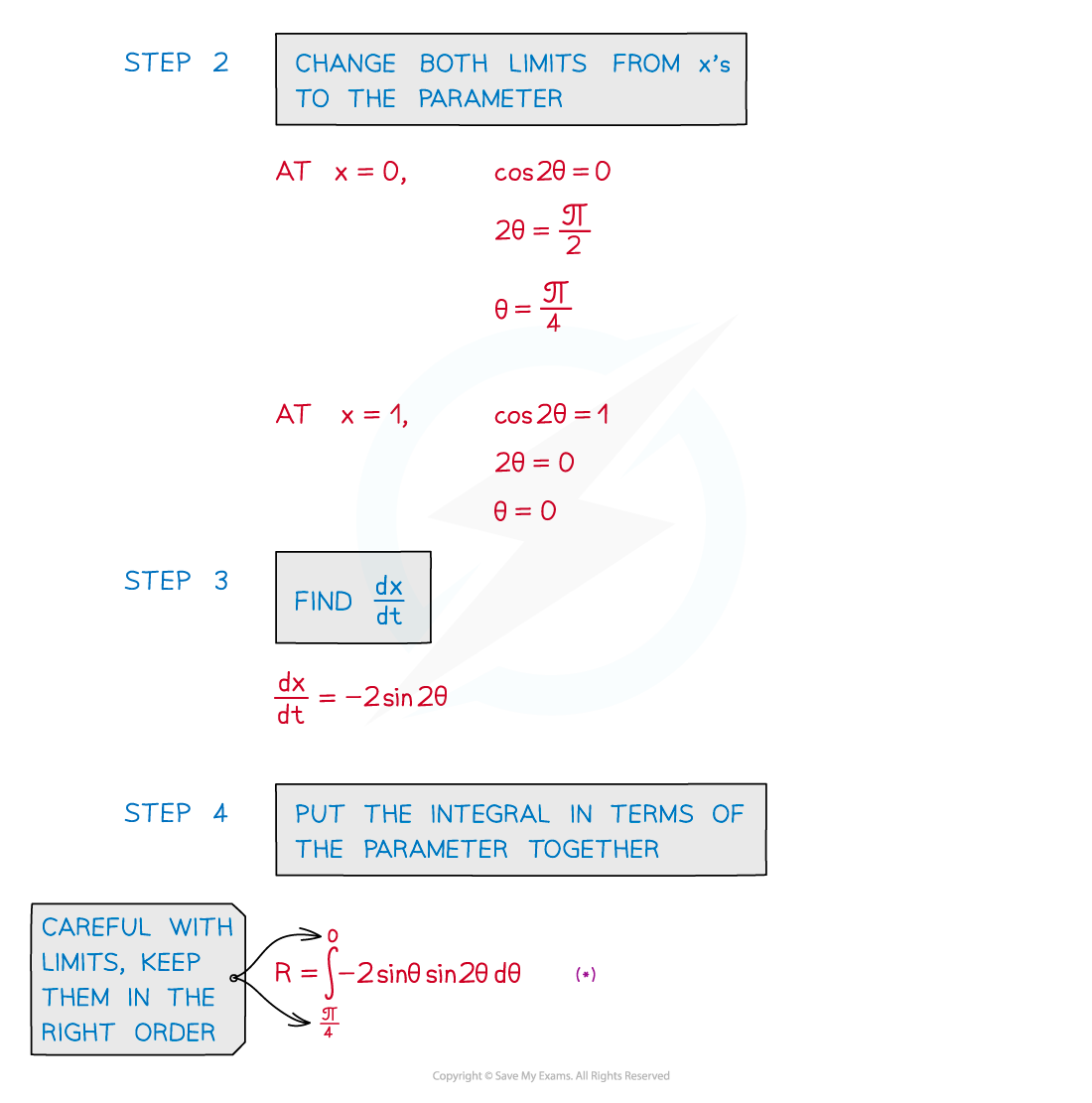#### Worked Example